Clutch Prep is now a part of Pearson
Ch.10 - Molecular Shapes & Valence Bond TheoryWorksheetSee all chapters

# VSEPR Theory (IGNORE)

See all sections
Sections
Valence Shell Electron Pair Repulsion Theory
Equatorial and Axial Positions
Electron Geometry
Molecular Geometry
Bond Angles
Hybridization
Molecular Orbital Theory
MO Theory: Homonuclear Diatomic Molecules
MO Theory: Heteronuclear Diatomic Molecules
MO Theory: Bond OrderJules Bruno

Valence shell electron pair repulsion (VSEPR) theory is a model for predicting the overall shape of a molecule. VSEPR theory postulates that a given molecule will adopt an ideal shape to reduce the repulsion between the surrounding elements and the lone pairs on the central element.

When drawing a compound you have to take into account two different systems for Lewis StructuresMolecular geometry and electronic geometry.

Molecular Geometry

A domain represents the number of surrounding elements and lone pairs on the central element. Under molecular geometry we treat surrounding elements as different from the lone pairs on the central element.

A = Central Element  X = Surrounding Element          E = Lone Pair (nonbonding electrons)

For example, a molecule with a designation of AX2E3 would mean it has a central element (A), two surrounding elements (X) and three lone pairs (E) on the central element.

Predicting Molecular Geometry

Based on molecular geometry when the domain is equal to 2 then AX2 is possible. This molecule would be linear and possess a bond angle of 180oMolecular Geometry (Domain of 2)

When the domain is equal to 3 then AX3 and AX2E1 are possible. As the central element gains lone pairs the idealized bond angle will be compressed. AX3 is trigonal planar and possesses an idealized bond angle of 120o, while AX2E1 is bent and has its bond angle compressed to less than 120o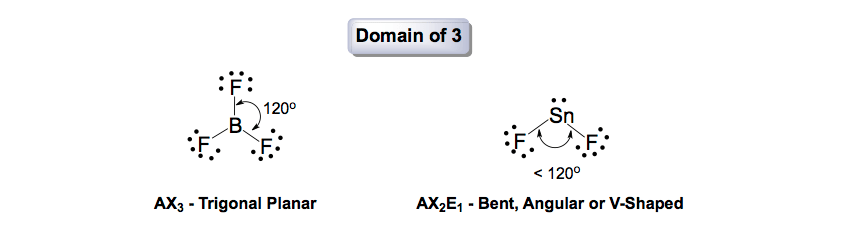Molecular Geometry (Domain of 3)

When the domain is equal to 4 then AX4, AX3Eand AX2E2 are possible. AX4 is tetrahedral, AX3E1 is trigonal pyramidal and AX2E2 is bent.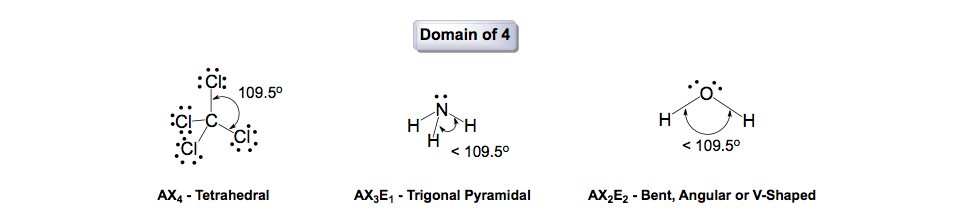Molecular Geometry (Domain of 4)

When the domain is equal to 5 then AX5, AX4E1, AX3E2, and AX2E3 are possible. AX5 is trigonal bipyramidal, AX4E1 is seesaw, AX3E2 is T-shaped and AX2E3 is linear.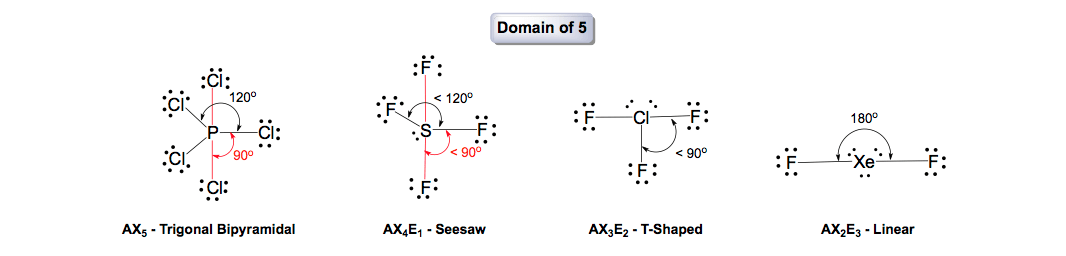Molecular Geometry (Domain of 5)

When the domain is equal to 6 then AX6, AX5E1, and AX4E2 are possible. AX6 is octahedral, AX5E1 is square pyramidal, and AX4E2 is square planar.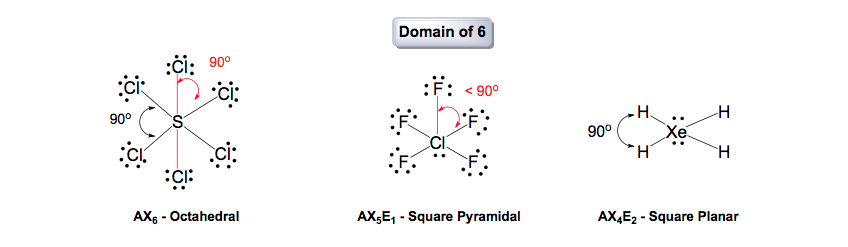Molecular Geometry (Domain of 6)

Electronic Geometry

Under electronic geometry we treat surrounding elements and lone pairs on the central element as the same.

A = Central Element      X = Surrounding Element & Lone Pairs on Central Element

For example, a molecule with a designation of AX2E3 would mean it has a central element (A), two surrounding elements (X) and three lone pairs (E) on the central element. Following its electronic geometry we would re-evaluate it as AX6.

Predicting Electronic Geometry

By treating surrounding elements and lone pairs on the central elements as the same we greatly reduce the number of shapes. However, notice that the compression of bond angles hasn’t changed because the behavior of lone pair repulsion still occurs.

Compounds with a domain of 2 are still seen as being linear.Electronic Geometry (Domain of 2)

Following the electronic geometry, all compounds with a domain of 3 are now seen as trigonal planar.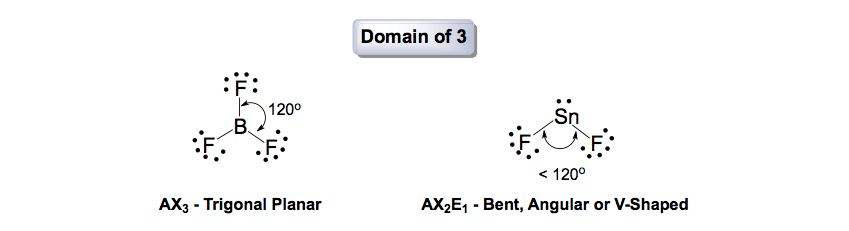Electronic Geometry (Domain of 3)

Compounds with a domain of 4 are tetrahedral.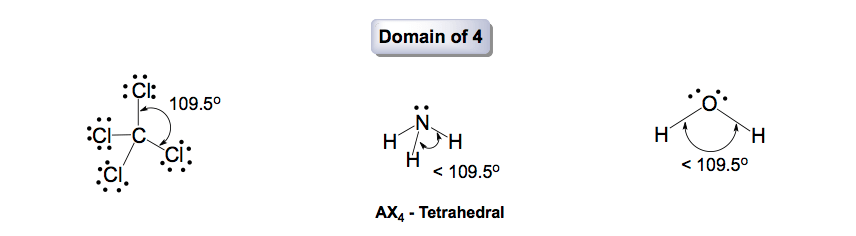Electronic Geometry (Domain of 4)

Compounds with a domain of 5 are trigonal bipyramidal.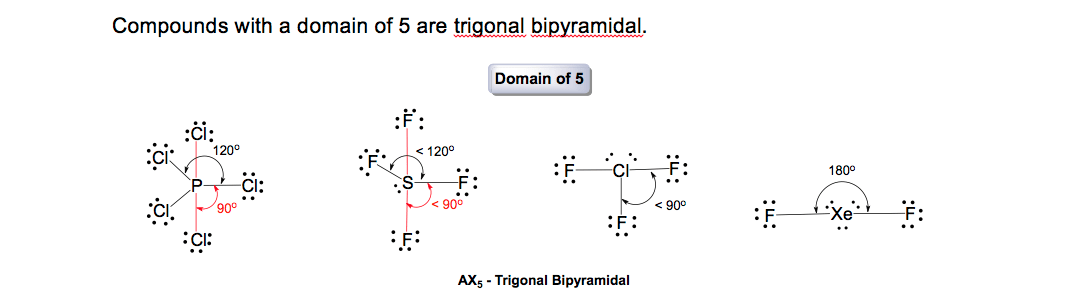Electronic Geometry (Domain of 5)

Compounds with a domain of 6 are octahedral.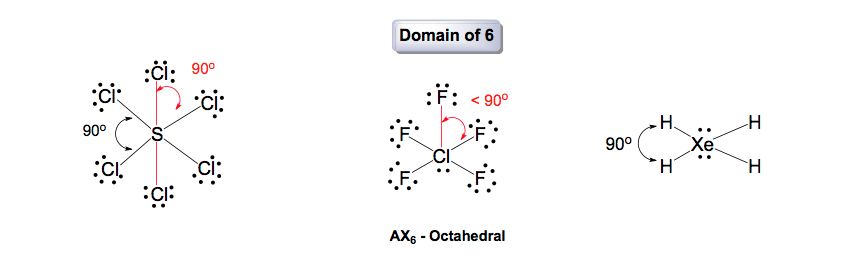Electronic Geometry (Domain of 6)

The electronic geometry can be seen as the simple geometry and is useful in determining hybridization (s, sp, sp2, sp3, sp3d, sp3d2)

More Than Just Shapes

The importance of these geometries goes beyond all the cool shapes. The overall polarity of a compound is based on both bond polarity and molecular shape. The way these molecules are formed is also based on our understanding of valence electrons, electron configurations and periodic trendsJules Bruno

Jules felt a void in his life after his English degree from Duke, so he started tutoring in 2007 and got a B.S. in Chemistry from FIU. He’s exceptionally skilled at making concepts dead simple and helping students in covalent bonds of knowledge.